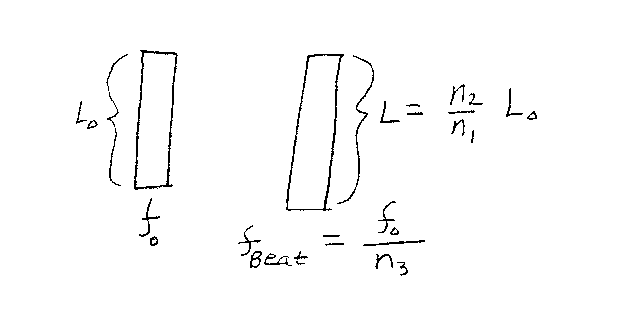Problem C10: Consider the two open-open pipes shown below. The one on the left has a length L0, and the one on the right has a slightly different length equal to L=(n2/n1)L0 where n1 and n2 are integers. The fundamental frequency of the pipe on the left is f0. If both pipes produce sound at the same time, what is the beat frequency of the combined sound? If the beat frequency is fbeat=f0/n3, what is n3? Note that all integers are unitless.n1 = n2 = Input n3:

If you are currently in my class, you can record your grade by entering your name and student ID number (without the leading zeros) below and clicking on "record grade".
 First Name = Last Name = ID = Problem: